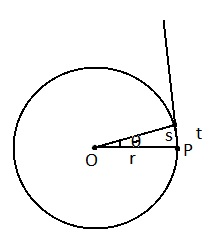Study Materials: CBSE Notes

Our CBSE Notes for Notes Motion In A Plane - Uniform Circular Motion | Class 11 Physics - Toppers Study is the best material for English Medium students cbse board and other state boards students.

Notes ⇒ Class 11th ⇒ Physics ⇒ Motion In A Plane

# Notes Motion In A Plane - Uniform Circular Motion | Class 11 Physics - Toppers Study

Topper Study classes prepares CBSE Notes on practical base problems and comes out with the best result that helps the students and teachers as well as tutors and so many ecademic coaching classes that they need in practical life. Our CBSE Notes for Notes Motion In A Plane - Uniform Circular Motion | Class 11 Physics - Toppers Study is the best material for English Medium students cbse board and other state boards students.

## Notes Motion In A Plane - Uniform Circular Motion | Class 11 Physics - Toppers Study

CBSE board students who preparing for class 11 ncert solutions maths and Physics solved exercise chapter Motion In A Plane available and this helps in upcoming exams 2023-2024.

### You can Find Physics solution Class 11 Chapter Motion In A Plane

• All Chapter review quick revision notes for chapter Motion In A Plane Class 11
• NCERT Solutions And Textual questions Answers Class 11 Physics
• Extra NCERT Book questions Answers Class 11 Physics
• Importatnt key points with additional Assignment and questions bank solved.

Chapter 0 Physics class 11

### Uniform Circular Motion class 11 Physics Chapter Motion In A Plane

• Notes Motion In A Plane - Uniform Circular Motion | Class 11 Physics - Toppers Study
• Class 11 Ncert Solutions
• Solution Chapter Motion In A Plane Class 11
• Solutions Class 11
• Chapter Motion In A Plane Uniform Circular Motion Class 11

## Notes Motion In A Plane - Uniform Circular Motion | Class 11 Physics - Toppers Study

Uniform Circular Motion

Circular motion: A point is moving in such a way that it keeps a contant distance from a fixed point, such motion is known as circular motion.P is the position of object.

O is the center which fixed point.

s is the linear displacement.

θ is the angular displacement.

t is the taken time in s displacement.

Angular displacement: The angle swept out by its radius vector in the given  time interval.

Angular velocity: The rate of change of angular velocity of a particle with respect to time is called its angular velocity. Which is denoted by Omega (ω).

According to rule of θ

θ = x/r

x = r . θ

Linear velocity (v) = x/t

Angular velocity (ω) = θ/t

instantinious velocity (ω) =

Time period: The time taken by a particle to complete one revolution along its circular path is called its period of revolution. It is denoted by Capital T and measured in second.

Frequency : The frequency of an object in a circular motion is define as the number of revolution completed in per unit time (one second). It is denoted by nu (v).

Relation between angular velocity (ω),  frequency (v) and time period (T) :

According to difinition of time period, a particle copmpletes one revolution in time T. Which traverses an angle of 2π radian in T second.

Angular velocity (ω) = θ/t = 2π/T

in term of nu (v)

(ω) = 2 πv

Important Study materials for classes 06, 07, 08,09,10, 11 and 12. Like CBSE Notes, Notes for Science, Notes for maths, Notes for Social Science, Notes for Accountancy, Notes for Economics, Notes for political Science, Noes for History, Notes For Bussiness Study, Physical Educations, Sample Papers, Test Papers, Mock Test Papers, Support Materials and Books.Mathematics Class - 11th

NCERT Maths book for CBSE Students.

books

## Study Materials List:

##### Notes ⇒ Class 11th ⇒ Physics
Physical world
Units and Measurement
Motion In A Straight Line
Motion In A Plane
Law of Motion
Work Energy and Power
Systems of Particles and Rotational Motion

New Books